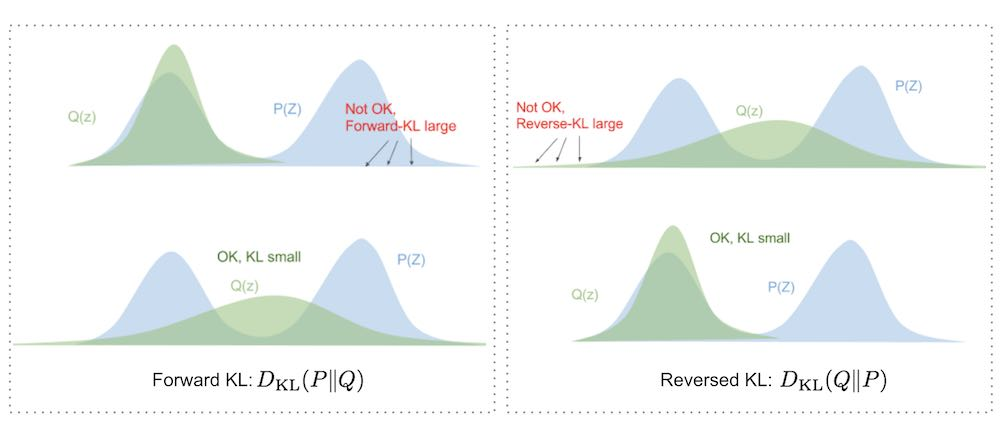# Kullback-leibler divergence

tags
Applied maths

## Definition

The KL divergence is not symmetric. For $$P, Q$$ defined on the same probability space $$\mathcal{X}$$, KL of $$Q$$ from $$P$$ is $KL(P, Q) = \sum_{x \in \mathcal{X}} P(x) \log\left( \frac{P(x)}{Q(x)} \right)$

It has two main interpretations:

• It is the information gain from using the right probability distribution $$P$$ instead of $$Q$$ or the amount of information lost by approximating $$P$$ with $$Q$$.
• The average difference in code length for a sequence following $$P$$ and using a code optimized for $$Q$$ to encode it.

## Forward and reverse KL

Eric Jang’s blog has an interesting visual explanation of the difference between forward and backward KL.Figure 1: Difference between forward and backward KL

So in summary, minimizing forward-KL “stretches” your variational distribution $$Q(Z)$$ to cover over the entire $$P(Z)$$ like a tarp, while minimizing reverse-KL “squeezes” the $$Q(Z)$$ under $$P(Z)$$.

## Links to this note

Last changed | authored by